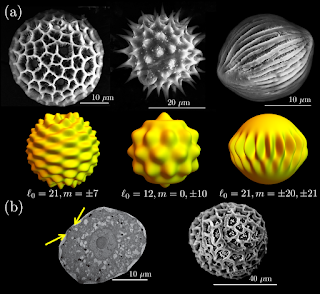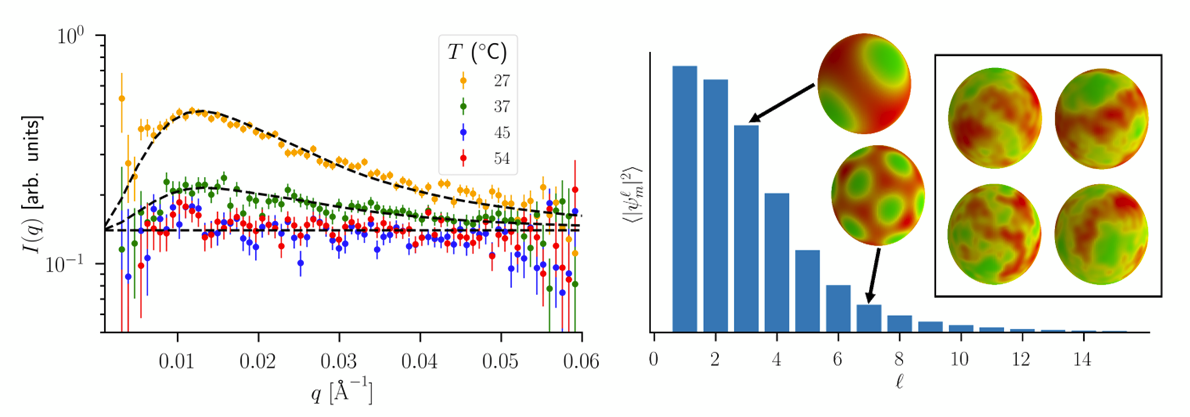### Pattern Formation

Patterns are found throughout nature. Our group is particularly interested in pattern formation on curved surfaces. Such patterns are typically frustrated in some way, where the pattern is unable to completely tile the surface. You might imagine such a situation if you are trying to wrap a striped wrapping paper around a basketball. The stripes will always have to terminate in some fashion, much like the lines of latitude and longitude on a globe. We are also interested in the molecular underpinnings of the patterns found in nature, which can be quite varied (see Why does nature create patterns? for an accessible take).

### Pollen grains

The outer coating of many organisms, including insect eggs, plant pollen, mite carapaces, and fungal spores, exhibits a wide range of intricate patterns.  These patterns have counter-intuitive features:  Although a single species reproduces the same pattern robustly, closely-related species may have wildly different patterns.  For example, in pollen (pictured below), the pattern may be used to uniquely identify a species.  We use physical approaches to try to understand the development of such patterns.(a) Scanning electron micrographs (SEMs) of plant pollen surfaces (top row, courtesy of A. Radja) are compared to the ordered states of a Brazovskii model on a sphere (bottom row), characterized by angular momentum modes $(\ell_0,m)$ (b) In the left panel, the undulating plasma membrane in a cross-section of pollen grain (a tunnelling electron microscope slice) is indicated by arrow.  In the right panel, the pollen outer surface is shown via SEM.
In pollen grains, the pattern template is likely formed via an undulated plasma membrane.  We modeled these undulations on the surface of a sphere, using a Brazovskii model. Our general approach interprets the pattern formation as a first-order phase transition to a patterned phase with some characteristic angular momentum mode $\ell_0$ on the surface of a sphere, corresponding to a pattern wavelength $\lambda_0 = 2 \pi R/\ell_0$ . Our theory yields predictions for the free energy difference $\Delta \Phi$ between an unpatterned, smooth state and a patterned state characterized by a scalar field $\Psi \equiv \Psi(\theta,\phi)$ describing, for instance, the amount of deposited material on the spherical pollen grain at longitude $\phi$ and colatitude $\theta$.  This scalar field is written as a linear combination of spherical harmonics $Y_{\ell}^m \equiv Y_{\ell}^m(\theta,\phi)$ with a particular $\ell_0$ and some choice of azimuthal quantum numbers $m$: $\Psi(\theta,\phi)=\sum_{m} \Psi_{\ell_0}^m Y_{\ell_0}^m$.  The computed free energy $\Delta \Phi$ (illustrated below), takes into account thermal fluctuations to the following Brazovskii Hamiltonian, perturbatively in the interaction $\mathcal{H}_{\mathrm{int}}$, which includes terms that are cubic and quadratic in the field $\Psi$: $\mathcal{H}[\Psi] = \frac{1}{2} \sum_{\ell,m} [K(\ell-\ell_0)^2+R^2 \tau]|\Psi_{\ell}^m|^2+ \mathcal{H}_{\mathrm{int}}.$
The pattern phases observed in pollen grains are similar to those found in phase-separated lipid vesicles with lipid compositions similar to those found in biological membranes. We are currently working on applying the theory to understand phase transitions in membranes and to see if these patterned phases have some biological relevance.

More recently, we examined how palynological data compares to our physical model and found that the theory can reproduce the majority of the observed pollen patterns .

## Lipid membranes

The heterogeneous mixture of lipids found in biological membranes provides an interesting playground for exploring the patterned phases. We look at model membranes consisting of various mixtures of common lipids: DSPC/DOPC/POPC. These mixtures, in the presence of cholesterol, phase separate if the temperature is sufficiently low. The different phases can induce different membrane curvatures, creating the possibility of generating the modulated phases found in pollen. Neutron scattering techniques may be used to identify this phase separation in small (nanoscopic) vesicles . We found that melatonin, a small molecule that associates with one of the lipid phases, can preserve the phase separation. Here are the fluorescently labelled domains at the micron scale:
Smaller, nanoscopic vesicles can be interrogated with neutron scattering techniques. We develop mathematical models that fit the scattering intensities in order to extract information about the domains. Here we fit our model to scattering data from phase separating lipid membranes, which exhibit a broad scattering peak as we lower the temperature below the phase separation phase transition:References
 M. O. Lavrentovich, E. M. Horsley, A. Radja, A. M. Sweeney, and R. D. Kamien First-order patterning transitions on a sphere as a route to cell morphology Proceedings of the National Academy of Sciences of the USA  113 5189 (2016)
  D. Bolmatov et al Deciphering melatonin-stabilized phase separation in phospholipid bilayers Langmuir 35 12236 (2019)
  A. Radja, E. M. Horsley, M. O. Lavrentovich, and A. M. Sweeney Pollen cell wall patterns form from modulated phases Cell 176, 856 (2019)